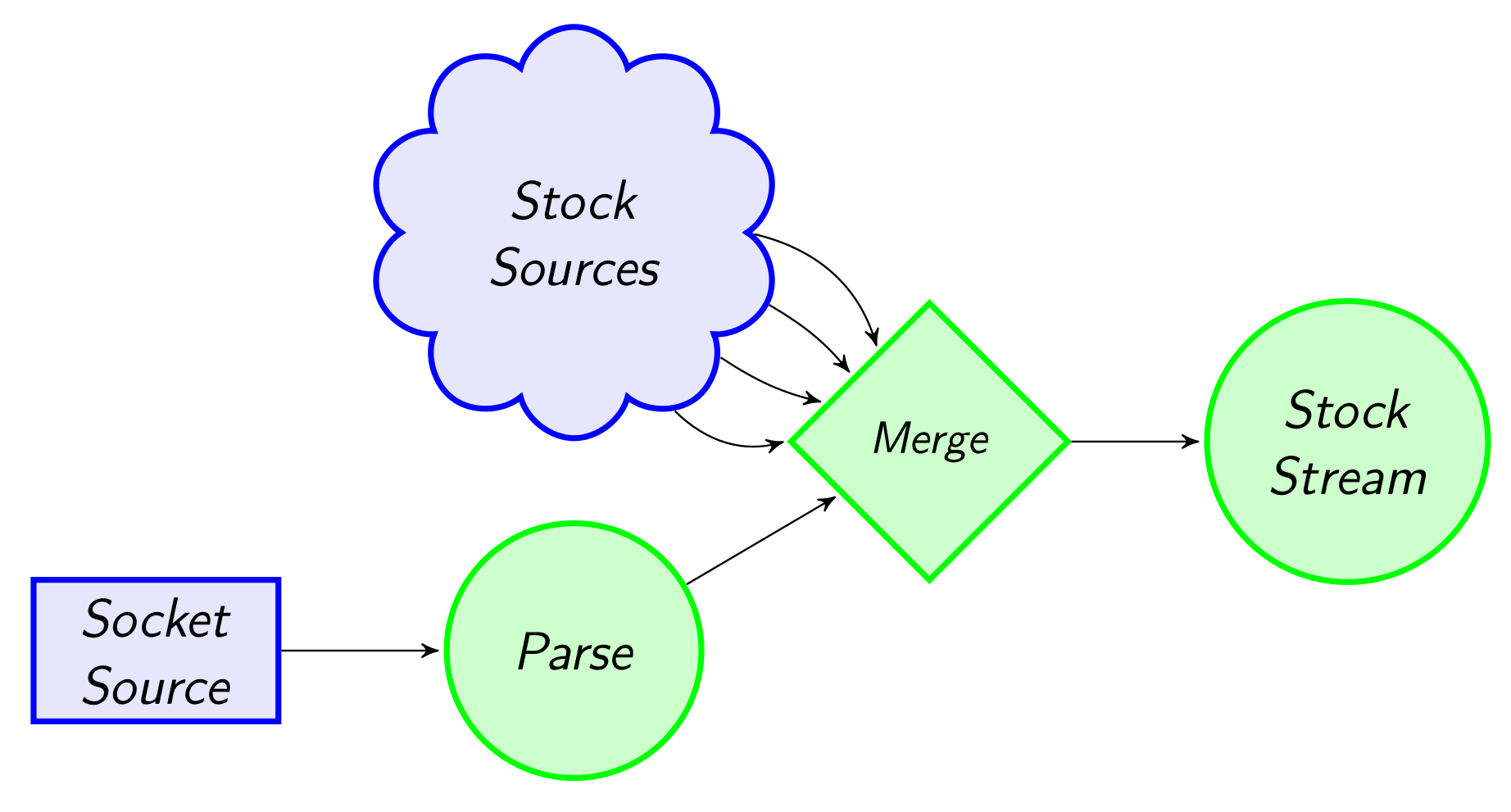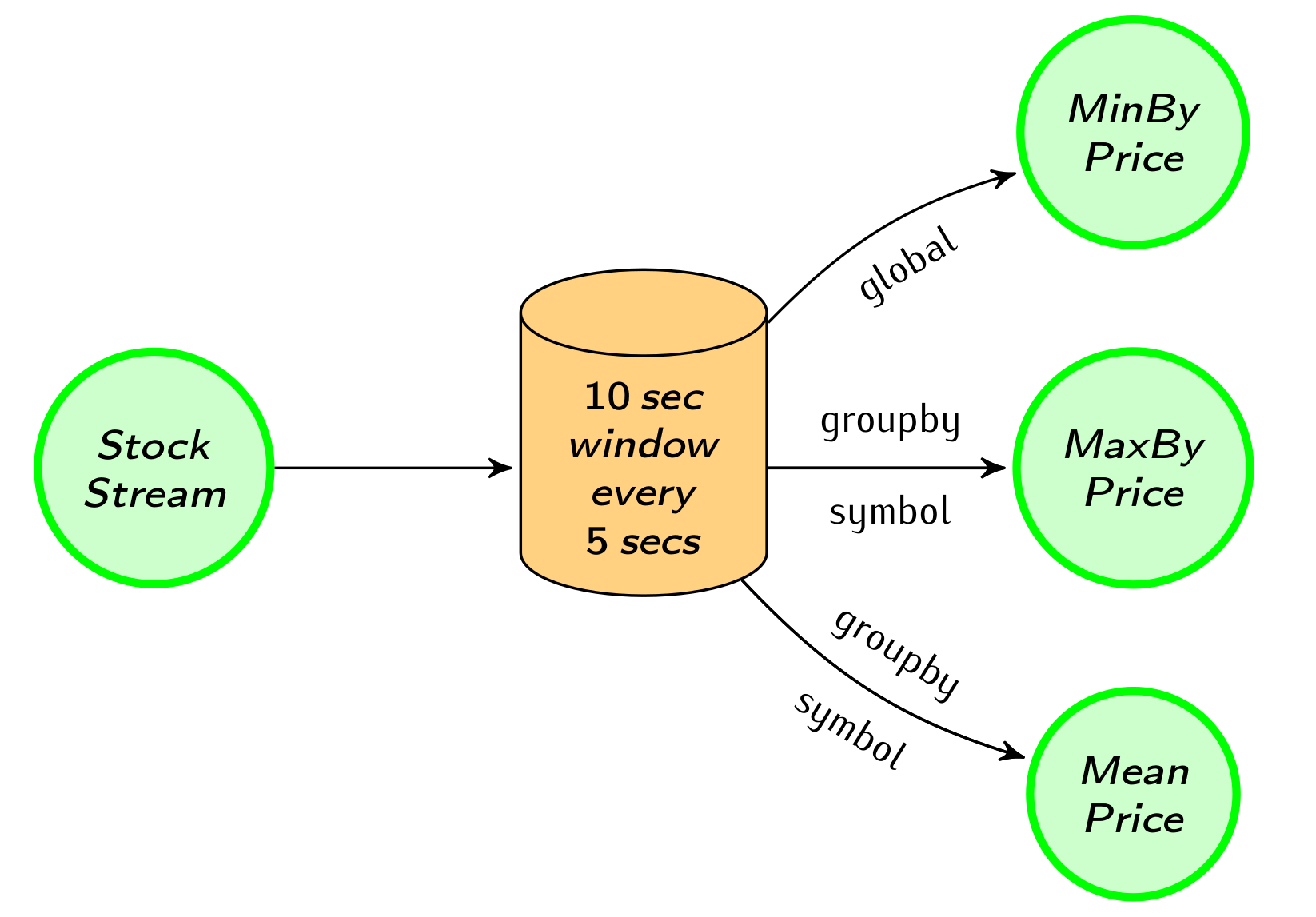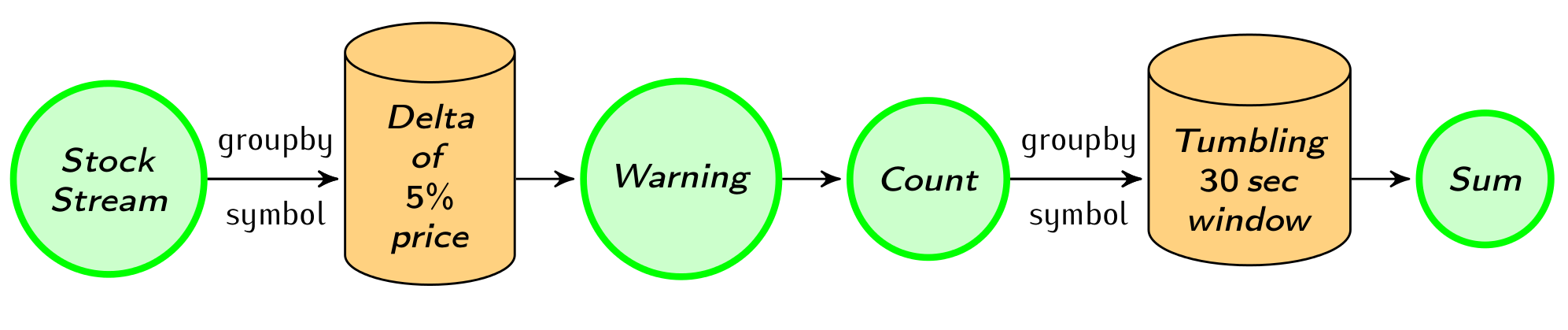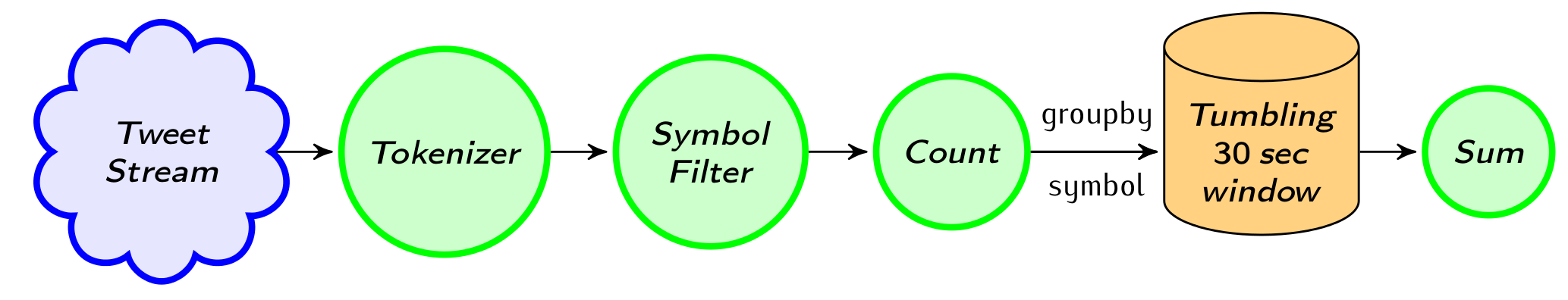09 Feb 2015

This post is the first of a series of blog posts on Flink Streaming, the recent addition to Apache Flink that makes it possible to analyze continuous data sources in addition to static files. Flink Streaming uses the pipelined Flink engine to process data streams in real time and offers a new API including definition of flexible windows.

In this post, we go through an example that uses the Flink Streaming API to compute statistics on stock market data that arrive continuously and combine the stock market data with Twitter streams. See the Streaming Programming Guide for a detailed presentation of the Streaming API.

First, we read a bunch of stock price streams and combine them into one stream of market data. We apply several transformations on this market data stream, like rolling aggregations per stock. Then we emit price warning alerts when the prices are rapidly changing. Moving towards more advanced features, we compute rolling correlations between the market data streams and a Twitter stream with stock mentions.

For running the example implementation please use the 0.9-SNAPSHOT version of Flink as a dependency. The full example code base can be found here in Scala and here in Java7.

First, let us create the stream of stock prices:

1. Read a socket stream of stock prices
2. Parse the text in the stream to create a stream of `StockPrice` objects
3. Add four other sources tagged with the stock symbol.
4. Finally, merge the streams to create a unified stream.``````def main(args: Array[String]) {

val env = StreamExecutionEnvironment.getExecutionEnvironment

//Read from a socket stream at map it to StockPrice objects
val socketStockStream = env.socketTextStream("localhost", 9999).map(x => {
val split = x.split(",")
StockPrice(split(0), split(1).toDouble)
})

//Generate other stock streams

//Merge all stock streams together
val stockStream = socketStockStream.merge(SPX_Stream, FTSE_Stream,
DJI_Stream, BUX_Stream)

stockStream.print()

env.execute("Stock stream")
}``````
``````public static void main(String[] args) throws Exception {

final StreamExecutionEnvironment env =
StreamExecutionEnvironment.getExecutionEnvironment();

//Read from a socket stream at map it to StockPrice objects
DataStream<StockPrice> socketStockStream = env
.socketTextStream("localhost", 9999)
.map(new MapFunction<String, StockPrice>() {
private String[] tokens;

@Override
public StockPrice map(String value) throws Exception {
tokens = value.split(",");
return new StockPrice(tokens,
Double.parseDouble(tokens));
}
});

//Generate other stock streams
DataStream<StockPrice> SPX_stream = env.addSource(new StockSource("SPX", 10));
DataStream<StockPrice> FTSE_stream = env.addSource(new StockSource("FTSE", 20));
DataStream<StockPrice> DJI_stream = env.addSource(new StockSource("DJI", 30));
DataStream<StockPrice> BUX_stream = env.addSource(new StockSource("BUX", 40));

//Merge all stock streams together
DataStream<StockPrice> stockStream = socketStockStream
.merge(SPX_stream, FTSE_stream, DJI_stream, BUX_stream);

stockStream.print();

env.execute("Stock stream");``````

See here on how you can create streaming sources for Flink Streaming programs. Flink, of course, has support for reading in streams from external sources such as Apache Kafka, Apache Flume, RabbitMQ, and others. For the sake of this example, the data streams are simply generated using the `generateStock` method:

``````val symbols = List("SPX", "FTSE", "DJI", "DJT", "BUX", "DAX", "GOOG")

case class StockPrice(symbol: String, price: Double)

def generateStock(symbol: String)(sigma: Int)(out: Collector[StockPrice]) = {
var price = 1000.
while (true) {
price = price + Random.nextGaussian * sigma
out.collect(StockPrice(symbol, price))
}
}``````
``````private static final ArrayList<String> SYMBOLS = new ArrayList<String>(
Arrays.asList("SPX", "FTSE", "DJI", "DJT", "BUX", "DAX", "GOOG"));

public static class StockPrice implements Serializable {

public String symbol;
public Double price;

public StockPrice() {
}

public StockPrice(String symbol, Double price) {
this.symbol = symbol;
this.price = price;
}

@Override
public String toString() {
return "StockPrice{" +
"symbol='" + symbol + '\'' +
", count=" + price +
'}';
}
}

public final static class StockSource implements SourceFunction<StockPrice> {

private Double price;
private String symbol;
private Integer sigma;

public StockSource(String symbol, Integer sigma) {
this.symbol = symbol;
this.sigma = sigma;
}

@Override
public void invoke(Collector<StockPrice> collector) throws Exception {
price = DEFAULT_PRICE;
Random random = new Random();

while (true) {
price = price + random.nextGaussian() * sigma;
collector.collect(new StockPrice(symbol, price));
}
}
}``````

To read from the text socket stream please make sure that you have a socket running. For the sake of the example executing the following command in a terminal does the job. You can get netcat here if it is not available on your machine.

``````nc -lk 9999
``````

If we execute the program from our IDE we see the system the stock prices being generated:

``````INFO    Job execution switched to status RUNNING.
INFO    Socket Stream(1/1) switched to SCHEDULED
INFO    Socket Stream(1/1) switched to DEPLOYING
INFO    Custom Source(1/1) switched to SCHEDULED
INFO    Custom Source(1/1) switched to DEPLOYING
…
1> StockPrice{symbol='SPX', count=1011.3405732645239}
2> StockPrice{symbol='SPX', count=1018.3381290039248}
1> StockPrice{symbol='DJI', count=1036.7454894073978}
3> StockPrice{symbol='DJI', count=1135.1170217478427}
3> StockPrice{symbol='BUX', count=1053.667523187687}
4> StockPrice{symbol='BUX', count=1036.552601487263}
``````

## Window aggregations

We first compute aggregations on time-based windows of the data. Flink provides flexible windowing semantics where windows can also be defined based on count of records or any custom user defined logic.

We partition our stream into windows of 10 seconds and slide the window every 5 seconds. We compute three statistics every 5 seconds. The first is the minimum price of all stocks, the second produces maximum price per stock, and the third is the mean stock price (using a map window function). Aggregations and groupings can be performed on named fields of POJOs, making the code more readable.``````//Define the desired time window
val windowedStream = stockStream
.window(Time.of(10, SECONDS)).every(Time.of(5, SECONDS))

//Compute some simple statistics on a rolling window
val lowest = windowedStream.minBy("price")
val maxByStock = windowedStream.groupBy("symbol").maxBy("price")
val rollingMean = windowedStream.groupBy("symbol").mapWindow(mean _)

//Compute the mean of a window
def mean(ts: Iterable[StockPrice], out: Collector[StockPrice]) = {
if (ts.nonEmpty) {
out.collect(StockPrice(ts.head.symbol, ts.foldLeft(0: Double)(_ + _.price) / ts.size))
}
}``````
``````//Define the desired time window
WindowedDataStream<StockPrice> windowedStream = stockStream
.window(Time.of(10, TimeUnit.SECONDS))
.every(Time.of(5, TimeUnit.SECONDS));

//Compute some simple statistics on a rolling window
DataStream<StockPrice> lowest = windowedStream.minBy("price").flatten();
DataStream<StockPrice> maxByStock = windowedStream.groupBy("symbol")
.maxBy("price").flatten();
DataStream<StockPrice> rollingMean = windowedStream.groupBy("symbol")
.mapWindow(new WindowMean()).flatten();

//Compute the mean of a window
public final static class WindowMean implements
WindowMapFunction<StockPrice, StockPrice> {

private Double sum = 0.0;
private Integer count = 0;
private String symbol = "";

@Override
public void mapWindow(Iterable<StockPrice> values, Collector<StockPrice> out)
throws Exception {

if (values.iterator().hasNext()) {s
for (StockPrice sp : values) {
sum += sp.price;
symbol = sp.symbol;
count++;
}
out.collect(new StockPrice(symbol, sum / count));
}
}
}``````

Let us note that to print a windowed stream one has to flatten it first, thus getting rid of the windowing logic. For example execute `maxByStock.flatten().print()` to print the stream of maximum prices of the time windows by stock. For Scala `flatten()` is called implicitly when needed.

## Data-driven windows

The most interesting event in the stream is when the price of a stock is changing rapidly. We can send a warning when a stock price changes more than 5% since the last warning. To do that, we use a delta-based window providing a threshold on when the computation will be triggered, a function to compute the difference and a default value with which the first record is compared. We also create a `Count` data type to count the warnings every 30 seconds.``````case class Count(symbol: String, count: Int)
val defaultPrice = StockPrice("", 1000)

//Use delta policy to create price change warnings
val priceWarnings = stockStream.groupBy("symbol")
.window(Delta.of(0.05, priceChange, defaultPrice))
.mapWindow(sendWarning _)

//Count the number of warnings every half a minute
val warningsPerStock = priceWarnings.map(Count(_, 1))
.groupBy("symbol")
.window(Time.of(30, SECONDS))
.sum("count")

def priceChange(p1: StockPrice, p2: StockPrice): Double = {
Math.abs(p1.price / p2.price - 1)
}

def sendWarning(ts: Iterable[StockPrice], out: Collector[String]) = {
}``````
``````private static final Double DEFAULT_PRICE = 1000.;
private static final StockPrice DEFAULT_STOCK_PRICE = new StockPrice("", DEFAULT_PRICE);

//Use delta policy to create price change warnings
DataStream<String> priceWarnings = stockStream.groupBy("symbol")
.window(Delta.of(0.05, new DeltaFunction<StockPrice>() {
@Override
public double getDelta(StockPrice oldDataPoint, StockPrice newDataPoint) {
return Math.abs(oldDataPoint.price - newDataPoint.price);
}
}, DEFAULT_STOCK_PRICE))
.mapWindow(new SendWarning()).flatten();

//Count the number of warnings every half a minute
DataStream<Count> warningsPerStock = priceWarnings.map(new MapFunction<String, Count>() {
@Override
public Count map(String value) throws Exception {
return new Count(value, 1);
}
}).groupBy("symbol").window(Time.of(30, TimeUnit.SECONDS)).sum("count").flatten();

public static class Count implements Serializable {
public String symbol;
public Integer count;

public Count() {
}

public Count(String symbol, Integer count) {
this.symbol = symbol;
this.count = count;
}

@Override
public String toString() {
return "Count{" +
"symbol='" + symbol + '\'' +
", count=" + count +
'}';
}
}

public static final class SendWarning implements MapWindowFunction<StockPrice, String> {
@Override
public void mapWindow(Iterable<StockPrice> values, Collector<String> out)
throws Exception {

if (values.iterator().hasNext()) {
out.collect(values.iterator().next().symbol);
}
}
}``````

## Combining with a Twitter stream

Next, we will read a Twitter stream and correlate it with our stock price stream. Flink has support for connecting to Twitter’s API but for the sake of this example we generate dummy tweet data.``````//Read a stream of tweets

//Extract the stock symbols
val mentionedSymbols = tweetStream.flatMap(tweet => tweet.split(" "))
.map(_.toUpperCase())
.filter(symbols.contains(_))

//Count the extracted symbols
val tweetsPerStock = mentionedSymbols.map(Count(_, 1))
.groupBy("symbol")
.window(Time.of(30, SECONDS))
.sum("count")

def generateTweets(out: Collector[String]) = {
while (true) {
val s = for (i <- 1 to 3) yield (symbols(Random.nextInt(symbols.size)))
out.collect(s.mkString(" "))
}
}``````
``````//Read a stream of tweets

//Extract the stock symbols
DataStream<String> mentionedSymbols = tweetStream.flatMap(
new FlatMapFunction<String, String>() {
@Override
public void flatMap(String value, Collector<String> out) throws Exception {
String[] words = value.split(" ");
for (String word : words) {
out.collect(word.toUpperCase());
}
}
}).filter(new FilterFunction<String>() {
@Override
public boolean filter(String value) throws Exception {
return SYMBOLS.contains(value);
}
});

//Count the extracted symbols
DataStream<Count> tweetsPerStock = mentionedSymbols.map(new MapFunction<String, Count>() {
@Override
public Count map(String value) throws Exception {
return new Count(value, 1);
}
}).groupBy("symbol").window(Time.of(30, TimeUnit.SECONDS)).sum("count").flatten();

public static final class TweetSource implements SourceFunction<String> {
Random random;
StringBuilder stringBuilder;

@Override
public void invoke(Collector<String> collector) throws Exception {
random = new Random();
stringBuilder = new StringBuilder();

while (true) {
stringBuilder.setLength(0);
for (int i = 0; i < 3; i++) {
stringBuilder.append(" ");
stringBuilder.append(SYMBOLS.get(random.nextInt(SYMBOLS.size())));
}
collector.collect(stringBuilder.toString());
}

}
}``````

## Streaming joins

Finally, we join real-time tweets and stock prices and compute a rolling correlation between the number of price warnings and the number of mentions of a given stock in the Twitter stream. As both of these data streams are potentially infinite, we apply the join on a 30-second window.``````//Join warnings and parsed tweets
val tweetsAndWarning = warningsPerStock.join(tweetsPerStock)
.onWindow(30, SECONDS)
.where("symbol")
.equalTo("symbol") { (c1, c2) => (c1.count, c2.count) }

val rollingCorrelation = tweetsAndWarning.window(Time.of(30, SECONDS))
.mapWindow(computeCorrelation _)

rollingCorrelation print

//Compute rolling correlation
def computeCorrelation(input: Iterable[(Int, Int)], out: Collector[Double]) = {
if (input.nonEmpty) {
val var1 = input.map(_._1)
val mean1 = average(var1)
val var2 = input.map(_._2)
val mean2 = average(var2)

val cov = average(var1.zip(var2).map(xy => (xy._1 - mean1) * (xy._2 - mean2)))
val d1 = Math.sqrt(average(var1.map(x => Math.pow((x - mean1), 2))))
val d2 = Math.sqrt(average(var2.map(x => Math.pow((x - mean2), 2))))

out.collect(cov / (d1 * d2))
}
}``````
``````//Join warnings and parsed tweets
DataStream<Tuple2<Integer, Integer>> tweetsAndWarning = warningsPerStock
.join(tweetsPerStock)
.onWindow(30, TimeUnit.SECONDS)
.where("symbol")
.equalTo("symbol")
.with(new JoinFunction<Count, Count, Tuple2<Integer, Integer>>() {
@Override
public Tuple2<Integer, Integer> join(Count first, Count second) throws Exception {
return new Tuple2<Integer, Integer>(first.count, second.count);
}
});

//Compute rolling correlation
DataStream<Double> rollingCorrelation = tweetsAndWarning
.window(Time.of(30, TimeUnit.SECONDS))
.mapWindow(new WindowCorrelation());

rollingCorrelation.print();

public static final class WindowCorrelation
implements WindowMapFunction<Tuple2<Integer, Integer>, Double> {

private Integer leftSum;
private Integer rightSum;
private Integer count;

private Double leftMean;
private Double rightMean;

private Double cov;
private Double leftSd;
private Double rightSd;

@Override
public void mapWindow(Iterable<Tuple2<Integer, Integer>> values, Collector<Double> out)
throws Exception {

leftSum = 0;
rightSum = 0;
count = 0;

cov = 0.;
leftSd = 0.;
rightSd = 0.;

//compute mean for both sides, save count
for (Tuple2<Integer, Integer> pair : values) {
leftSum += pair.f0;
rightSum += pair.f1;
count++;
}

leftMean = leftSum.doubleValue() / count;
rightMean = rightSum.doubleValue() / count;

//compute covariance & std. deviations
for (Tuple2<Integer, Integer> pair : values) {
cov += (pair.f0 - leftMean) * (pair.f1 - rightMean) / count;
}

for (Tuple2<Integer, Integer> pair : values) {
leftSd += Math.pow(pair.f0 - leftMean, 2) / count;
rightSd += Math.pow(pair.f1 - rightMean, 2) / count;
}
leftSd = Math.sqrt(leftSd);
rightSd = Math.sqrt(rightSd);

out.collect(cov / (leftSd * rightSd));
}
}``````